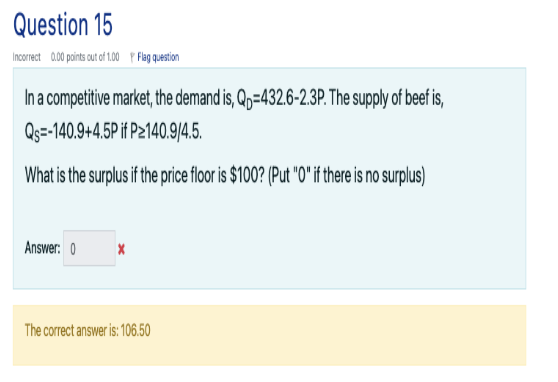# (Solved): How did they get the answer 106.50? In a competitive market, the demand is, \( Q_{D}=432.6-2.3 P ...How did they get the answer 106.50?

In a competitive market, the demand is, \( Q_{D}=432.6-2.3 P \). The supply of beef is, \( Q_{S}=-140.9+4.5 P \) if \( P \geq 140.9 / 4.5 \) What is the surplus if the price floor is \( \\$ 100 \) ? (Put "0" if there is no surplus) Answer: The correct answer is: \( 106.50 \)

We have an Answer from Expert